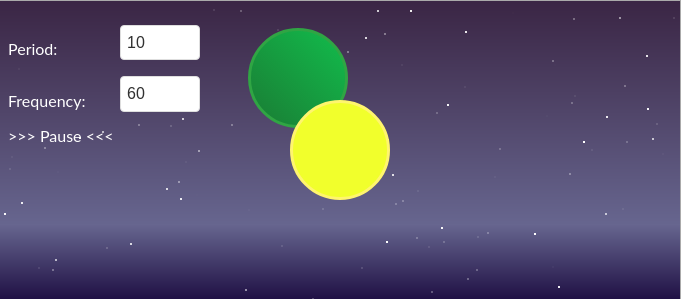Example fcf-example-planetfcf-example-planet.zip

Repository:
https://github.com/fcfdev/fcf-example-planet

The first application and introduction to the templates

Description

An introductory example of using templates in the FCF framework. This example introduces the basics of template design and the use of arguments. Requirements

For the example, a version of the FCF framework is required at least 1.1.48

Start-up instruction